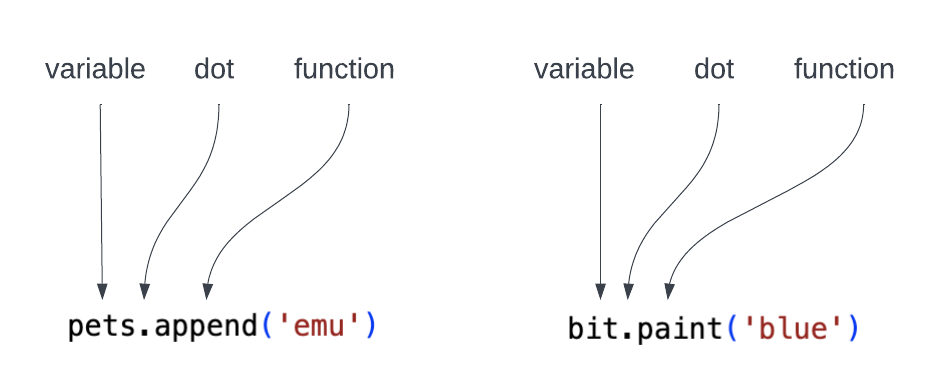Computer Science

# Lists

A list contains a collection of values. You have already seen variables that hold a single value:

``number = 5``

A variable that references a list can hold multiple values, in order:

``````names = ['Maria', 'Mariano', 'Anna', 'Antonino']
numbers = [5, 8, 10, 13, 42]``````A list can hold as many values as you want. We can even have an empty list:

``twilight_books_i_like = []``

## Length

To get the length of a list, use `len`:

``number_of_names = len(names)``

Here, `number_of_names` will be equal to 4.

## Appending to a list

One of the many functions we can perform on a list is appending items to it. This means adding items to the end of the list. For example:

``````pets = ['cat', 'horse', 'dog']
pets.append('emu')``````

This will result in the pets list now containing `['cat', 'horse', 'dog', 'emu']`.

Note the syntax here — variable dot function:You can use input loops to start with an empty list and then add more items to it. In the zip file you will find a small program in `get_names.py` that illustrates how to do this:

``````if __name__ == '__main__':
names = []
while True:
name = input("Give me a name: ")
if name == 'q':
break
names.append(name)

print(names)``````
• We start with an empty list, using `names = []`.

• We use the event stream pattern, looping forever and then breaking out of the loop if the person enters ‘q’ instead of a name. (Note, if you were making a program to enter characters in Star Trek, you might not want to do it this way.)

• We use `append()` to add each name to the list.

If you run this program, you will see something like this:

``````Give me a name: me
Give me a name: you
Give me a name: everyone
Give me a name: q
['me', 'you', 'everyone']``````

## Iteration

Once you have a list, you can iterate (or loop) through it using `for ... in`:

``````for number in numbers:
print(number)``````

Each time through the `for` loop, Python sets the variable `number` to one of the numbers in the list, in order. Since we have set the `numbers` variable to `[5, 8, 10, 13, 42]`, the first time through the loop, `number = 5`. The second time through the loop, `number = 8`, and so forth. So this code will print:

``````5
8
10
13
42``````

The name of the variable can be whatever you like. For example:

``````for cute_puppies in numbers:
print(cute_puppies)``````

However, you want to be sure that your friends can read your code and make sense of it!

Your code is much more readable if you use variables that make sense.